# Plotting Expression Profiles

## Description

Plotting Expression Profiles

When using NMF for clustering in particular, one looks for strong associations between the basis and a priori known groups of samples. Plotting the profiles may highlight such patterns.

## Usage

```profplot(x, ...)

S3 (default)
`profplot`(x, y, scale = FALSE, match.names = TRUE, legend = TRUE, confint = TRUE,
Colv, labels, annotation, ..., add = FALSE)```

## Arguments

x
a matrix or an NMF object from which is extracted the mixture coefficient matrix. It is extracted from the best fit if `x` is the results from multiple NMF runs.
y
a matrix or an NMF object from which is extracted the mixture coefficient matrix. It is extracted from the best fit if `y` is the results from multiple NMF runs.
scale
a logical that specifies whether the columns of the matrices should be scaled into proportions (i.e. to sum up to one) before plotting. Default is `FALSE`.
match.names
a logical that indicates if the profiles in `y` should be subset and/or re-ordered to match the profile names in `x` (i.e. the rownames). This is attempted only when both `x` and `y` have names.
legend
a logical that specifies whether drawing the legend or not, or coordinates specifications passed to argument `x` of `legend`, that specifies the position of the legend.
confint
logical that indicates if confidence intervals for the R-squared should be shown in legend.
Colv
specifies the way the columns of `x` are ordered before plotting. It is used only when `y` is missing. It can be:
• a single numeric value, specifying the index of a row of `x`, that is used to order the columns by ```x[, order(x[abs(Colv),])]```. Decreasing order is specified with a negative index.
• an integer vector directly specifying the order itself, in which case the columns are ordered by `x[, Colv]`
• a factor used to order the columns by `x[, order(Colv)]` and as argument `annotation` if this latter is missing or not `NA`.
• any other object with a suitable `order` method. The columns are by ```x[, order(Colv)]```
labels
a character vector containing labels for each sample (i.e. each column of `x`). These are used for labelling the x-axis.
annotation
a factor annotating each sample (i.e. each column of `x`). If not missing, a coloured raw is plotted under the x-axis and annotates each sample accordingly. If argument `Colv` is a factor, then it is used to annotate the plot, unless `annotation=NA`.
...
graphical parameters passed to `matplot` or `matpoints`.
logical that indicates if the plot should be added as points to a previous plot

## Details

The function can also be used to compare the profiles from two NMF models or mixture coefficient matrices. In this case, it draws a scatter plot of the paired profiles.

## Examples

``````
# create a random target matrix
v <- rmatrix(50, 10)

# fit a single NMF model
res <- nmf(v, 3)
profplot(res)
``````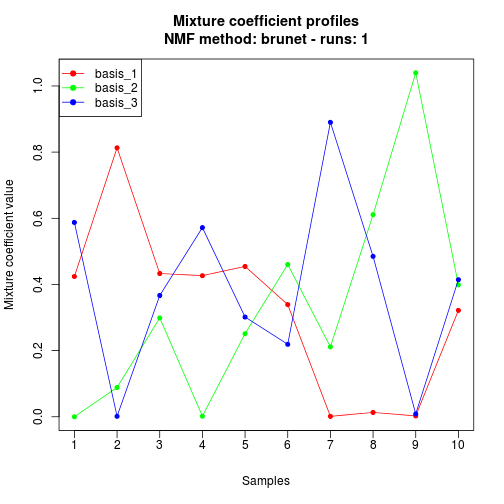``````
# ordering according to first profile
profplot(res, Colv=1) # increasing
``````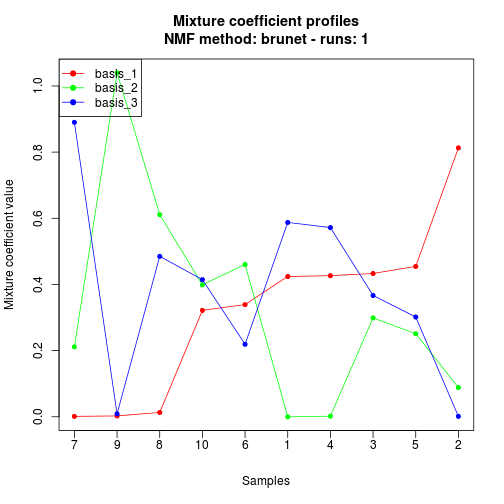``````profplot(res, Colv=-1) # decreasing
``````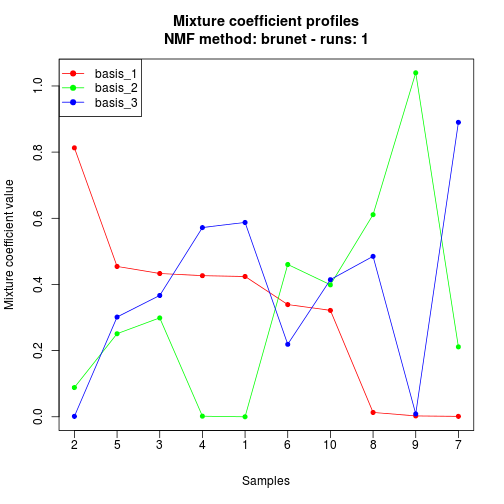``````
# fit a multi-run NMF model
res2 <- nmf(v, 3, nrun=3)
profplot(res2)
``````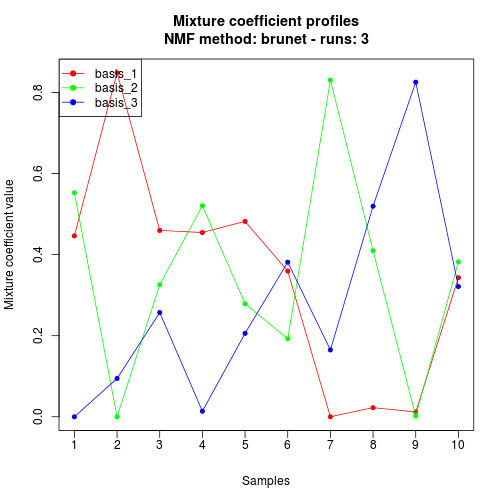``````
# draw a profile correlation plot: this show how the basis components are
# returned in an unpredictable order
profplot(res, res2)
``````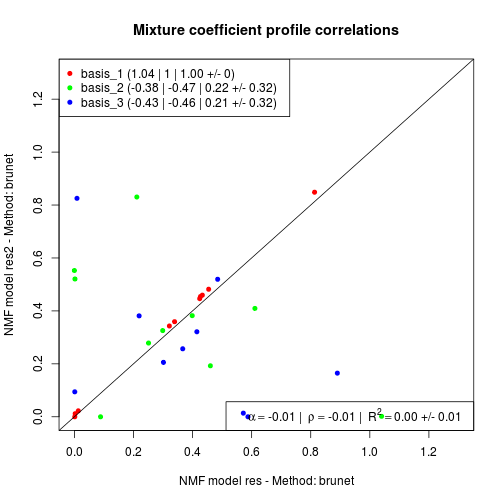``````
# looking at all the correlations allow to order the components in a "common" order
profcor(res, res2)
``````
``````##         [,1]    [,2]    [,3]
## [1,]  0.9999 -0.3571 -0.6357
## [2,] -0.6441 -0.4674  0.9988
## [3,] -0.3815  0.9986 -0.4605
``````
``````
``````

`profcor`